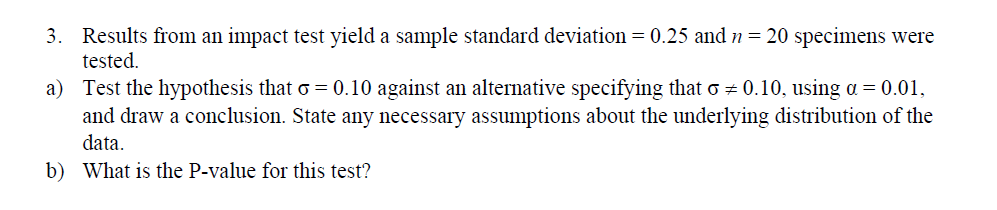# 3. Results from an impact test yield a sample standard deviation 0.25 and-20 specimens wereicsted.Test the hypothesis that σ-0.10 against an alternative specifying that σand draw a conclusion. State any necessary assumptions about the underlying distribution of thedataWhat is the P-value for this test?a)0.10. using α0.01.b)

Question
22 viewshelp_outlineImage Transcriptionclose3. Results from an impact test yield a sample standard deviation 0.25 and-20 specimens were icsted. Test the hypothesis that σ-0.10 against an alternative specifying that σ and draw a conclusion. State any necessary assumptions about the underlying distribution of the data What is the P-value for this test? a) 0.10. using α 0.01. b) fullscreen
check_circle

Step 1

Chi-square test us usually used to test population variance.

Given s= 0.25 and n= 20

Null Hypothesis: Population standard deviation = 0.1

Alternative Hypothesis: Population standard deviation is not equal to 0.1

Now we can calculate the chi-square test statistic using the formula below

Step 2

Let's calculate the critical value using alpha = 0.01 and degrees of freedom = n-1 = 19

Critical Value = 36.191

As we ...

### Want to see the full answer?

See Solution

#### Want to see this answer and more?

Solutions are written by subject experts who are available 24/7. Questions are typically answered within 1 hour.*

See Solution
*Response times may vary by subject and question.
Tagged in

### Hypothesis Testing